## Link functions for binary data

Back to course page

Based on the distribution function of the standard logistic distribution.

$g(\mu)=\frac{\mu}{1-\mu}$

$g^{-1}(\eta)=\frac{\exp(\eta)}{1+\exp(\eta)}$

#### Plot of the inverse logistic link function: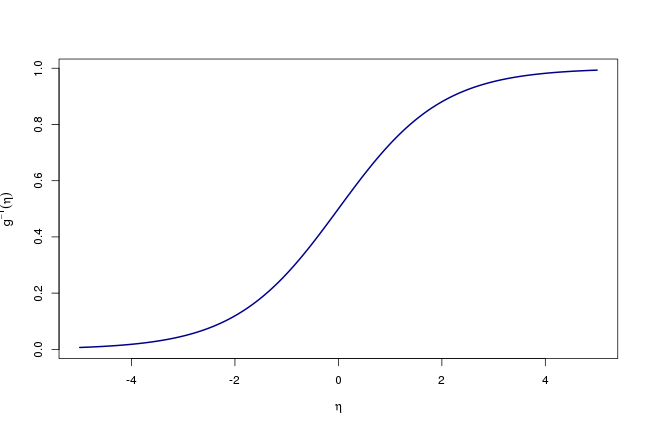Based on the distribution function of the standard normal distribution.

$g(\mu)=\Phi^{-1}(\mu)$

$g^{-1}(\eta)=\Phi(\eta)$

#### Plot of the inverse probit link function: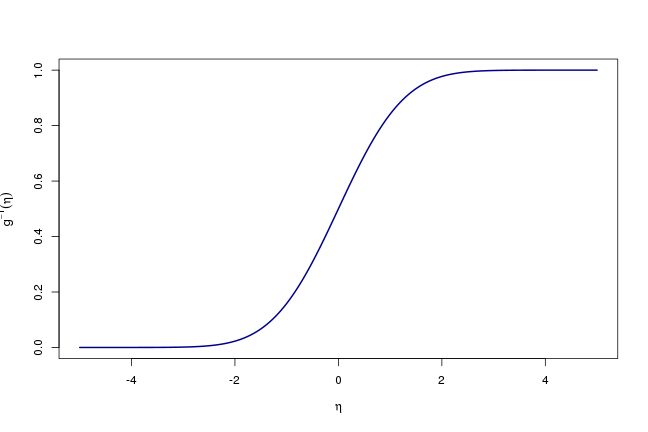Based on the distribution function of the standard Cauchy distribution.

$g(\mu)=\frac{1}{\pi}\arctan(\mu)+\frac{1}{2}$

$g^{-1}(\eta)=\tan[\pi(\eta-\frac{1}{2})]$

#### Plot of the inverse cauchit link function: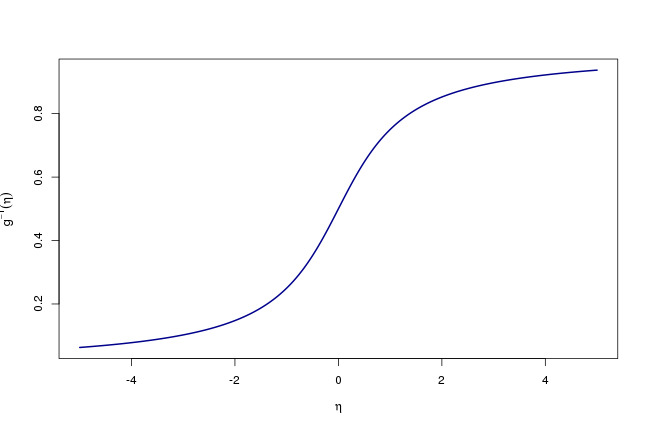Related to the distribution function of the extreme value (Gumbel) distribution. The link is not symmetric.

$\quad g(\mu)=\log(-\log(1-\mu))$

$g^{-1}(\eta)=1-\exp(-\exp(\eta))$

#### Plot of the inverse complementary log-log link function: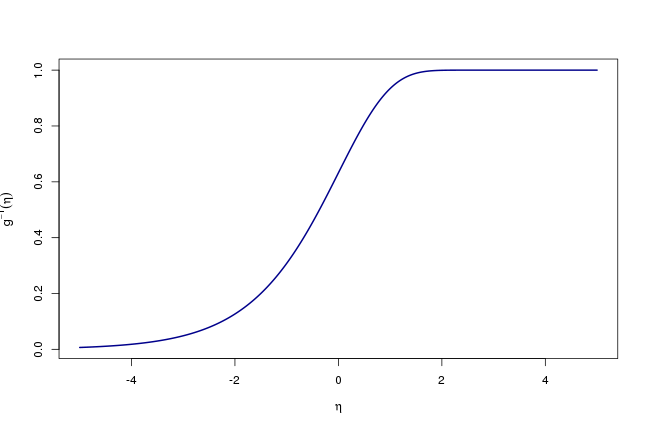## Comparison of inverse link functions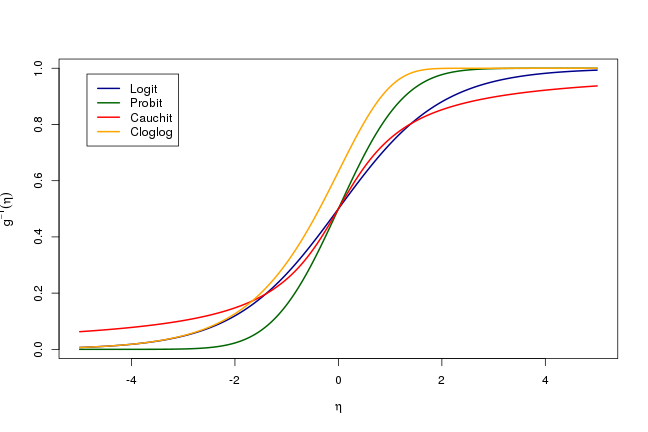Back to course page